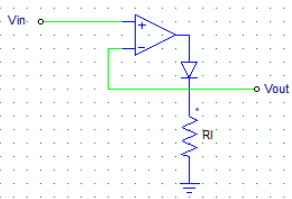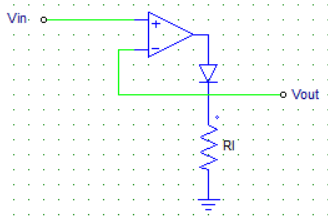# Test: Types of Power Diodes

## 20 Questions MCQ Test Power Electronics | Test: Types of Power Diodes

Description
Attempt Test: Types of Power Diodes | 20 questions in 20 minutes | Mock test for Electrical Engineering (EE) preparation | Free important questions MCQ to study Power Electronics for Electrical Engineering (EE) Exam | Download free PDF with solutions
QUESTION: 1

### Shown below is the diagram of an ideal super diode. When the input voltage Vin is negative, then the output voltage Vout = ?​Solution:

When the input voltage is negative, there would be a negative voltage on the diode so it works like an open circuit. Hence no current flows through the load and Vout is zero.

QUESTION: 2

### In order to reduce the reverse recovery time of the diodes, __________ is carried out.

Solution:

Platinum & gold doping improves the performance of the devices.

QUESTION: 3

### Which of the following diodes uses a metal-semiconductor junction?

Solution:

Schottky diode uses a Al-Semiconductor junction.

QUESTION: 4

Which of the below mentioned statements is false regarding Schottky diodes?

Solution:

The majority charge carriers in a Schottky diode are electrons not holes.

QUESTION: 5

A Schottky diode _____

Solution:

Due to the metal-silicon junction there are no stored charges hence, no reverse recovery time, due to which the switching is faster.

QUESTION: 6

Which of the following are/is the majority charge carriers in a Schottky diode?

Solution:

The metal has no holes hence major(almost full) current flows due to the electrons only.

QUESTION: 7

In a Schottky diode, the silcon is usually

Solution:

Usually only n-type silicon is used because the p-type has certain limitations.

QUESTION: 8

As compared to a p-n junction diode(of the same rating), a Schottky diode has ___________

Solution:

Due to the metal-silicon junction there are no stored charges, hence no reverse recovery time due to which the switching is faster.

QUESTION: 9

A Schottky diode has __________

Solution:

Schottky diode uses a Al-Semiconductor junction.

QUESTION: 10

A Schottky diode can be switchd off much faster than an equivalent p-n junction diode due to its

Solution:

Due to the metal-silicon junction there are no stored charges, hence no reverse recovery time due to which the switching is faster.

QUESTION: 11

Ideally the voltage drop across a conducting diode must be

Solution:

Ideal conduction = No losses.

QUESTION: 12

When reverse breakdown occurs in a diode

Solution:

Recall the I-V curve of a diode in the 3rd quadrant.

QUESTION: 13

Schottky diodes are also called as

Solution:

Due to the metal used to carry the current, it is also called as a hot carrier diode.

QUESTION: 14

In a Schottky diode, the aluminum metal acts as a __________

Solution:

The Al (Metal) always acts as the anode.

QUESTION: 15

If the doping levels of the semiconductor is increased, then the width of the depletion layer

Solution:

Higher the doping, more the number of charge carrier available to neutralize the opposite charges on the junction.

QUESTION: 16

As compared to a p-n junction device of equal rating, the Schottky diode has

Solution:

Low reverse voltage rating is the only drawback against a p-n junction diode.

QUESTION: 17

In a Schottky diode, the silicon layer acts as a _____________

Solution:

The metal acts as the anode and the semiconductor as a cathode.

QUESTION: 18

In a certain power electronics application, it is required that the voltage at the load terminals is to be kept within a certain range of voltages only. Among the device listed below, which would be the most ideal choice for this application?

Solution:

Zener diode is used as a voltage regulating device.

QUESTION: 19

Shown below is the diagram of an ideal super diode. When the input voltage Vin is positive, then the output voltage Vout = ?​Solution:

Input is positive, hence it is amplified by the operational amplifier which switches the diode on. Current flows through the load & because of the feedback, the output voltage is equal to the input voltage.

QUESTION: 20

Zener diodes allow a current to flow in the reverse direction, when the

Solution:

Zener diode has voltage regulating property. When voltage reaches above a certain value (Zener voltage), current starts to flow in the reverse direction.Use Code STAYHOME200 and get INR 200 additional OFF Use Coupon Code